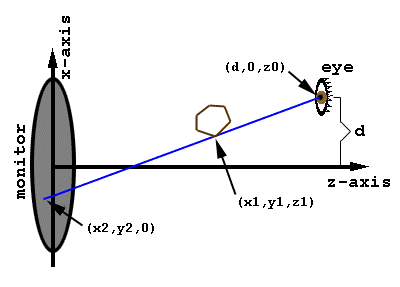## Geometry of Stereo 3D Projection

This page was created in response to several requests for details on how the hypercube applet projects a point from 3-space onto the 2-dimensional surface of the monitor.Figure: projection from an arbitrary 3D point (x1,y1,z1) to a 2D point on the monitor (looking down from above the monitor).

### Notation:

Points in 3-dimensional space are represented by triples of numbers or symbols enclosed in parentheses, e.g. "(x1,y1,z1)". The first number or symbol represents the x-coordinate, the second represents the y-coordinate, the third represents the z-coordinate.

### Features of the Figure:

• The monitor: the center of the monitor surface is the "origin" of the coordinate system. The monitor surface lies in the x-y plane (all points on the monitor surface have a z-coordinate of zero).
• A brown hexagon: a 3D figure to be projected onto the monitor.
• A person's right eye, located at (d,0,z0). The separation between the right eye and the left eye (not shown) is 2d. The eyes are at a distance z0 from the monitor and vertically centered (the y-coordinate is zero).
• A blue ray of light which originates at a point on the monitor surface (x2,y2,0), passes through a vertex (x1,y1,z1) of the hexagon, and enters the right eye.

### Overview of the Math:

The 2 points (x1,y1,z1) and (d,0,z0) determine a straight line. We need to find the point (x2,y2,0) where that line touches the plane of the monitor. The first step is to get a formula for the line. Then we find the point on the line where the z-coordinate is zero.

### The Math

It is easy to verify that the following is a "parametric" representation of the line:
```x = t * x1 + (1 - t) * d
y = t * y1 + (1 - t) * 0
z = t * z1 + (1 - t) * z0
```
where the asterisk (*) represents multiplication.

The "parameter" is "t". By varying "t", you can make (x,y,z) equal any point on the line. It is easy to see that if t = 1, parametric representation gives (x,y,z) = (x1,y1,z1). If t = 0, the parametric representation gives (x,y,z) = (d,0,z0). Thus we have verified that the line passes through the necessary two points.

Now we need to find out which value of "t" gives a z-coordinate of zero (i.e. a point in the plane of the monitor). So we set z=0 in the 3rd equation:

```0 = t * z1 + (1 - t) * z0
```
Solve for "t":
```t = z0 / (z0 - z1)
```
Plug this value of "t" into the equations for "x" and "y" to get the intersection (x2,y2,0) of the line with the screen.
```x2 = (z0 * x1 - z1 * d) / (z0 - z1)
y2 = (z0 * y1) / (z0 - z1)
```
This is the desired result. For the left eye, replace "d" with "-d".